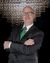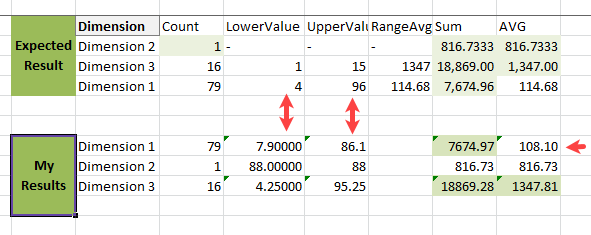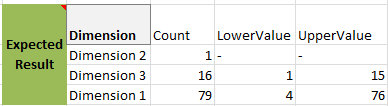# App Development

Discussion board where members can learn more about Qlik Sense App Development and Usage.

Announcements
QlikWorld Online 2021, May 10-12: Our Free, Virtual, Global Event REGISTER TODAY
cancel
Showing results for
Search instead for
Did you mean:Contributor II

## Power BI formula translate to Qlik Sense

Dear All,

I need advice on how to make a Power bi formula work in Qlik Sense. I have been trying to come up with something similar in qlik sense for quite some time now, but it never provided the flexability i had in Power Bi.

I need to apply percentile to a data set and be able to change dinamicly. If the % i am applying is 5% of 100 rows = 5rows, i need it to take the same 5% percentile when i filter for example on 50 rows and get 2.5 rows and so on.

Here is the formula in BI that worked:

TTR =
VAR LowerValue = CALCULATE(PERCENTILE.INC ('Table'[Index], .03), ('Table'))
VAR UpperValue = CALCULATE(PERCENTILE.INC ( 'Table'[Index], .95 ), ('Table'))

RETURN
CALCULATE(AVERAGE('Table'[Time]), AND('Table'[Index] >= LowerValue,'Table'[Index] <= UpperValue))

Really appreciate if anyone has any ideas.
Best regards,
Julia
11 RepliesEmployee

In Qlik you can create variables with values, or expressions like you need either inside the Load Script, or in the UI. Variables can be assigned and calculated at a later time via what is called \$ sign expansion or calculated with a fixed value right then.

SET vLiteral = 1 + 1; // This stores the string "1 + 1";

LET vSum = 1 + 1; // This stores 2

The Dynamic nature of whether you want 5% of the rows, or 9% of the rows can also be done through a variable and then you can use a Variable Input chart type to use an input box or slider etc to set that value.

Calculating an aggregate would be done using what is called Set Analysis in Qlik. Syntax is different than PowerBI but essentially the same.

There is a previous Qlik Community post that demonstrates the syntax you are looking for regarding a range of values. https://community.qlik.com/t5/QlikView-App-Dev/Set-Analysis-Compare-Number-with-Range-Classification...Contributor II
Author

Hi Dalton_Ruer,

Thank you for the reply.

I did play a bit with the range formulas and here is what i came up with:

IF(NUM(RANK(TOTAL -SUM([Resolution Time]),4))>=fractile(TOTAL aggr(Rank(total [Case Number],4,0),[Case Number]), 0.05)

and

NUM(RANK(TOTAL -SUM([Resolution Time]),4))<=fractile(TOTAL aggr(Rank(total [Case Number],4,0),[Case Number]), 0.95),

Avg( [Resolution Time]),

NULL())

Unfortunately it srill doesnt work in a table.

I am attaching sample data with my table results as well.

Thank you in advance,

JuliaSpecialist II

Thank you for sharing a sample file, I tried to understand your expected result with no joy; my Qlik application is returning the same figures you posted, but I failed to replicate your expected results with your Excel data, as I explain below:My Lower and Upper values are way different to yours, your Dimension 1's are close to my Dimension 3's which confused me; still there are some differences; I don't know where your Dimension 3's 1 and 15 are coming from.

These are the Excel formulas I am using:

``````Lower Value: PERCENTILE.INC(\$A82:\$A97,0.05)
Upper Value: PERCENTILE.INC(\$A82:\$A97,0.95)``````

Where the range: A82 - A97 correspond with the dimension 3, calculating the percentile on the Case Number column.

As I could be wrong with my formula, would you please include the formulas you are using? or your figures were taken directly from PowerBI?

If you took the figures from PowerBI, notice that Excel returns a different result for the same expression.

Once you clarify my doubts and confusions I will be able to help you better.

Hope this helps,

Arnaldo Sandoval
A journey of a thousand miles begins with a single step.Contributor II
Author

Hi Arnaldo Sandoval,

Thank you for looking into the data.

Probably i should have explained it better, my expected result table is calculated in excel (i added 2 new sheets in my file for explanation). In order to calculated the result i separate my Dimensions and looking just at the data set by itself, than i create a flag which shows where my lower and upper percentiles are, like 1 and 15, everything in-between is my result. That is what i expect to happen in QlikSense and this is how it works in Power Bi.

Hope that clarifies it.

Thanks, JuliaSpecialist II

Thank you for the updated Excel file, it helped but you haven't answer my question about the formulas your are using to get the Lower and Upper Values in the Excel file, your original post includes formulas that I believe were taken from Power BI, anyhow.

Comments on the new Excel file:

• The sheet All include the field (column) Case Number, which is a unique sequential number for all your data, e.g. Case Number equal 2 belong to Dimension 3 and nobody else.
• Your new sheets Dimension 3 and Dimension 1 also feature the Case Number field (column) and they are unique sequential number for each Dimension, e.g. Case Number equal 2 appears on the Dimension 1 and Dimension 3 sheets; in other words, this field is different to the Case Number on the sheet All.
• We can't have a field (column) name with two different meanings, because "Case Number" in the sheet All does not represent the same meaning as "Case Number" on sheets: Dimension 1 and Dimension 3; for this reason, the Qlik Application (attached) introduced a new field (column) named RT_Seq, it replaces your second Case Number field (column) appearing on the sheets Dimension 1 and Dimension 3

Load Script:

``````UserData:
LOAD
"Case Number"        As Case_Number,
"Resolution Time"    As Resolution_Time,
"Dimension"          As Dimension,
AutoNumber("Case Number", "Dimension") As RT_Seq,
"IF(
NUM(RANK(TOTAL -SUM([Resolution Time]),4))>=fractile(TOTAL aggr(Rank(total [Case Number],4,0),[Case Number]), 0.05)
and
NUM(RANK(TOTAL -SUM([Resolution Time]),4))<=fractile(TOTAL aggr(Rank(total [Case Number],4,0),[Case Number]), 0.95),1,0)"  As Formula_1,
"IF(NUM(RANK(TOTAL -SUM([Resolution Time]),4))>=fractile(TOTAL aggr(Rank(total [Case Number],4,0),[Case Number]), 0.05)" As Formula_2,
"Count"              As Count
FROM [lib://SampleData/Percentile-Sample-data.xlsx]
(ooxml, embedded labels, table is Sheet1);``````

The last three fields in the Load statement were not required for the solution, so the simplified script is:

``````UserData:
LOAD
"Case Number"        As Case_Number,
"Resolution Time"    As Resolution_Time,
"Dimension"          As Dimension,
AutoNumber("Case Number", "Dimension") As RT_Seq
FROM [lib://SampleData/Percentile-Sample-data.xlsx]
(ooxml, embedded labels, table is Sheet1);``````

Note: I am loading Sheet1 from the first Excel file you shared.

The column RT_Seq

``    AutoNumber("Case Number", "Dimension") As RT_Seq``

This field generates a "sequential" number similar to the "Case Number" in your second Excel file, but we can't use the same name for the reason given above.

User Interface / Front End:

I added this table on the UI/Front End of the solution.• Here, It shows your three dimensions, and on its last two columns, the fields: Lower Value and Upper Value, the remaining columns are informative.
• The Count(Case_Number) (Notice I replaced the space in Case Number with an underscore to avoid the double quotes, which are a nuisance if we need that fields to create Set Analysis expressions, which could be the case)
• The Count(Case_Number) match the Max(RT_Seq) which is good, these fields Min(RT_Seq) and Max(RT_Seq) define the range of values for each dimension.
• I read some articles explaining PERCENTILES, but none of them elaborates on what happens if the set of data (Count(Case_Number)) is equal to 1, so, in such cases (Dimension 2) it returned a dash character.
• I wont explain here on this reply how I calculated Lower Value and Upper Value because I am still waiting for your answer, I could write that I took some executive decisions for the lack of information; we can speak about these two fields once I know more about the logic behind them in your Excel file.

References: (Articles/topics I read working out this reply)

• Percentiles  Good article, informative.
• Percentiles: Interpretations and Calculations  Another good article, but the one above was more informative.
• Percentile  This Wikipedia page was quite good as well.
• Fractile While trying to find the definitions for Fractile and Percentiles because Qlik feature a Fractile function while Power BI/Excel use Percentile, I discovered that statisticians do not agree on a definition 🤣
• PercentileINC Excel/Power BI explanation of the percentile function, it was not helpful.
• Fractile Qlik's Fractile help page, I took its example, and it is part of the attached solution.

Final Comments:

The attached solution does not implement the fields Sum and AVG as, you guess, I am waiting confirmation on the Lower and Upper values calculations.

Hope this helps,

Arnaldo Sandoval
A journey of a thousand miles begins with a single step.Contributor II
Author

Hi Arnaldo Sandoval,

Thank you for reply, i am still to test and read all of it. In the mean time here are my formulas for the Lower and Upper value:

LowerValue = fractile(TOTAL aggr(Rank(total [Case Case Number],4,0),[Case Case Number]), 0.05)

UpperValue = fractile(TOTAL aggr(Rank(total [Case Case Number],4,0),[Case Case Number]), 0.95)

And you are right, i should have used a separate field for indexing the data and not just ajust the Case number one. Thats how i did it in Power BI.

Thanks again, i will let you know when i review the rest.

JuliaSpecialist II

I think you misunderstood my question, as I believe the formulas you posted are Qlik Sense's formulas, when I am after the Excel or Power BI ones, my internet search for a function 'Fractile' in Excel/PBI returned no hits. I need them because in the attached solution I forced the Lower and Upper values, which won't be a good/solid base to go ahead with the rest of the solution.

Regards,

Arnaldo Sandoval
A journey of a thousand miles begins with a single step.Contributor II
Author

Hi,

The formula in my original post was from Power Bi and in excel i just calculate the % from the Total Case count *5% or 95%.

Here is the Power bi as well:

TTR =
VAR LowerValue = CALCULATE(PERCENTILE.INC ('Table'[Index], .03), ('Table'))
VAR UpperValue = CALCULATE(PERCENTILE.INC ( 'Table'[Index], .95 ), ('Table'))

RETURN
CALCULATE(AVERAGE('Table'[Time]), AND('Table'[Index] >= LowerValue,'Table'[Index] <= UpperValue))

I dont have anything else....

Hope that helps.

Best regards,

JuliaSpecialist II

Thanks @Julia_Velinova

I used those formulas with your Excel sample, my Excel does not support the CALCULATE function, and this is what I got;These are the formulas for Lower/Upper values:

``````=PERCENTILE.INC(A2:A80,0.05)
=PERCENTILE.INC(A2:A80,0.95)``````

While your expected results rounded those value in ways that I did not understand, because your results are:The Lower Value seems to be a truncation of the results returned by the PERCENTILE.INC function, while the rule for the Upper Value is not.

Anyhow, I think that I now why the Upper Value was truncated for Dimension 3 and rounded up for Dimension 1.

I will write out the rounding rules for these fields and implement the SET analysis in the Qlik Application sample for your problem; I will do that in my next reply.

Hope this helps,

Arnaldo Sandoval
A journey of a thousand miles begins with a single step.